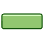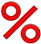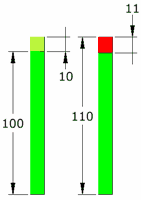# Percentage Change

Subtract the old from the new, then divide by the old value. Show that as a Percentage.

## Comparing Old to NewChange: subtract old value from new value. Example: You had 5 books, but now have 7. The change is: 7−5 = 2.Percentage Change: show that change as a percent of the old value ... so divide by the old value and make it a percentage: So the percentage change from 5 to 7 is: 2/5 = 0.4 = 40%

Percentage Change is all about comparing old to new values. See percentage change, difference and error for other options.

## How to Calculate

Here are two ways to calculate a percentage change, use the one you prefer:

### Method 1

 Step 1: Calculate the change (subtract old value from the new value) Step 2: Divide that change by the old value (you will get a decimal number) Step 3: Convert that to a percentage (by multiplying by 100 and adding a "%" sign) Note: when the new value is greater then the old value, it is a percentage increase, otherwise it is a decrease.

### Method 2

 Step 1: Divide the New Value by the Old Value (you will get a decimal number) Step 2: Convert that to a percentage (by multiplying by 100 and adding a "%" sign) Step 3: Subtract 100% from that Note: when the result is positive it is a percentage increase, if negative, just remove the minus sign and call it a decrease.

## Examples

### Example: A pair of socks went from \$5 to \$6, what is the percentage change?

• Step 1: \$5 to \$6 is a \$1 increase
• Step 2: Divide by the old value: \$1/\$5 = 0.2
• Step 3: Convert 0.2 to percentage: 0.2×100 = 20% rise.

• Step 1: Divide new value by old value: \$6/\$5 = 1.2
• Step 2: Convert to percentage: 1.2×100 = 120% (i.e. \$6 is 120% of \$5)
• Step 3: Subtract 100%: 120% − 100% = 20%, and that means a 20% rise.### Another Example: There were 160 smarties in the box yesterday, but now there are 116, what is the percentage change?

Answer (Method 1): 160 to 116 is a decrease of 44. Compared to yesterday's value: 44/160 = 0.275 = 27.5% decrease.

Answer (Method 2): Compare today's value with yesterday's value: 116/160 = 0.725 = 72.5%, so the new value is 72.5% of the old value.
Subtract 100% and you get −27.5%, or a 27.5% decrease.

## Why Compare to Old Value?

Because you are saying how much a value has changed.

### Example: Milk was \$2, now it is \$3, did it rise \$1 compared to \$2 or \$3 ?

We compare to the original \$2 value, so we say the change is \$1/\$2 = 0.5 which is a 50% increase.

## The Formula

You can also put the values into this formula:

New Value − Old Value |Old Value| × 100%

(The "|" symbols mean absolute value, so negatives become positive)

### Example: There were 200 customers yesterday, and 240 today:

240 − 200 |200| × 100% = 40 200 × 100% = 20%

A 20% increase.

### Example: But if there were 240 customers yesterday, and 200 today we would get:

200 − 240 |240| × 100% = −40 240 × 100% = −16.6...%

A 16.6...% decrease.

## How to Reverse a Rise or Fall

Some people think that a percentage increase can be "reversed" by the same percentage decrease. But no!

### Example: 10% of 100

A 10% increase from 100 is an increase of 10, which equals 110 ...

... but a 10% reduction from 110 is a reduction of 11 (10% of 110 is 11)

So we ended up at 99 (not the 100 we started with)What happened?

• 10% took us up 10
• Then 10% took us down 11

Because the percentage rise or fall is in relation to the old value:

• The 10% increase was applied to 100
• But the 10% decrease was applied to 110

### How to do it properly

To "reverse" a percentage rise or fall, use the right formula here:

To Reverse: Use this Percent: Example 10%
An "x" percent rise:
x/(1+x/100)
10/(1+10/100) = 10/(1.1) = 9.0909...
An "x" percent fall:
x/(1−x/100)
10/(1−10/100) = 10/(0.9) = 11.111...

 Or use this handy-dandy calculator (just type in a value and click in the other box) Percent Rise: <=> Percent Fall: